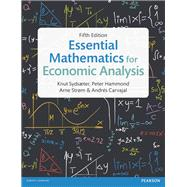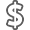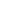# Essential Mathematics for Economic Analysis

• ISBN13:

• ISBN10:

## 1292074612

• Edition: 5th
• Format: Paperback
• Publisher: Pearson

Note: Supplemental materials are not guaranteed with Rental or Used book purchases.

Purchase Benefits

•Free Shipping On Orders Over \$35!
Your order must be \$35 or more to qualify for free economy shipping. Bulk sales, PO's, Marketplace items, eBooks and apparel do not qualify for this offer.
•Get Rewarded for Ordering Your Textbooks! Enroll Now
•We Buy This Book Back!
In-Store Credit: \$23.89
Check/Direct Deposit: \$22.75
PayPal: \$22.75
List Price: \$86.65 Save up to \$58.94
• Rent Book \$43.33Free Shipping

TERM
PRICE
DUE
IN STOCK USUALLY SHIPS IN 24 HOURS.
*This item is part of an exclusive publisher rental program and requires an additional convenience fee. This fee will be reflected in the shopping cart.

### Supplemental Materials

What is included with this book?

• The New copy of this book will include any supplemental materials advertised. Please check the title of the book to determine if it should include any access cards, study guides, lab manuals, CDs, etc.
• The Used, Rental and eBook copies of this book are not guaranteed to include any supplemental materials. Typically, only the book itself is included. This is true even if the title states it includes any access cards, study guides, lab manuals, CDs, etc.

### Summary

Were you looking for the book with access to MyMathLab Global? This product is the book alone and does NOT come with access to MyMathLab Global. Buy Essential Mathematics for Economic Analysis, 5th edition, with MyMathLab Global access card (ISBN 9781292074719) if you need access to MyMathLab Global as well, and save money on this resource. You will also need a course ID from your instructor to access MyMathLab Global.

ESSENTIAL MATHEMATICS FOR ECONOMIC ANALYSIS

Fifth Edition

An extensive introduction to all the mathematical tools an economist needs is provided in this worldwide bestseller.

“The scope of the book is to be applauded” Dr Michael Reynolds, University of Bradford

“Excellent book on calculus with several economic applications” Mauro Bambi, University of York

New to this edition:

• The introductory chapters have been restructured to more logically fit with teaching.
• Several new exercises have been introduced, as well as fuller solutions to existing ones.
• More coverage of the history of mathematical and economic ideas has been added, as well as of the scientists who developed them.
• New example based on the 2014 UK reform of housing taxation illustrating how a discontinuous function can have significant economic consequences.
• The associated material in MyMathLab has been expanded and improved.

Knut Sydsaeter was Emeritus Professor of Mathematics in the Economics Department at the University of Oslo, where he had taught mathematics for economists for over 45 years.

Peter Hammond is currently a Professor of Economics at the University of Warwick, where he moved in 2007 after becoming an Emeritus Professor at Stanford University. He has taught mathematics for economists at both universities, as well as at the Universities of Oxford and Essex.

Arne Strom is Associate Professor Emeritus at the University of Oslo and has extensive experience in teaching mathematics for economists in the Department of Economics there.

Andrés Carvajal is an Associate Professor in the Department of Economics at University of California, Davis.

Sydsaeter Essential Mathematics for Economic Analysis – 5e TOC

Ch01: Essentials of Logic and Set Theory

1.1 Essentials of set theory

1.2 Some aspects of logic

1.3 Mathematical proofs

1.4 Mathematical induction

Ch02: Algebra

2.1 The real numbers

2.2 Integer powers

2.3 Rules of algebra

2.4 Fractions

2.5 Fractional powers

2.6 Inequalities

2.7 Intervals and absolute values

2.8 Summation

2.9 Rules for sums

2. 10 Newton’s binomial formula

2. 11 Double sums

Ch03: Solving Equations

3.1 Solving equations

3.2 Equations and their parameters

3.4 Nonlinear equations

3.5 Using implication arrows

3.6 Two linear equations in two unknowns

Ch04: Functions of One Variable

4.1 Introduction

4.2 Basic definitions

4.3 Graphs of functions

4.4 Linear functions

4.5 Linear models

4.7 Polynomials

4.8 Power functions

4.9 Exponential functions

4. 10 Logarithmic functions

Ch05: Properties of Functions

5.1 Shifting graphs

5.2 New functions from old

5.3 Inverse functions

5.4 Graphs of equations

5.5 Distance in the plane

5.6 General functions

Ch06: Differentiation

6.1 Slopes of curves

6.2 Tangents and derivatives

6.3 Increasing and decreasing functions

6.4 Rates of change

6.5 A dash of limits

6.6 Simple rules for differentiation

6.7 Sums, products and quotients

6.8 The Chain Rule

6.9 Higher-order derivatives

6. 10 Exponential functions

6. 11 Logarithmic functions

Ch07: Derivatives in Use

7.1 Implicit differentiation

7.2 Economic examples

7.3 Differentiating the inverse

7.4 Linear approximations

7.5 Polynomial approximations

7.6 Taylor's formula

7.7 Elasticities

7.8 Continuity

7.9 More on limits

7. 10 The intermediate value theorem and Newton’s method

7. 11 Infinite sequences

7. 12 L'Hôpital's Rule

Ch08: Single-Variable Optimization

8.1 Extreme points

8.2 Simple tests for extreme points

8.3 Economic examples

8.4 The Extreme Value Theorem

8.5 Further economic examples

8.6 Local extreme points

8.7 Inflection points

Ch09: Integration

9.1 Indefinite integrals

9.2 Area and definite integrals

9.3 Properties of definite integrals

9.4 Economic applications

9.5 Integration by parts

9.6 Integration by substitution

9.7 Infinite intervals of integration

9.8 A glimpse at differential equations

9.9 Separable and linear differential equations

Ch10: Topics in Financial Mathematics

10.1   Interest periods and effective rates

10.2   Continuous compounding

10.3   Present value

10.4   Geometric series

10.5   Total present value

10.6   Mortgage repayments

10.7   Internal rate of return

10.8   A glimpse at difference equations

Ch11: Functions of Many Variables

11.1   Functions of two variables

11.2   Partial derivatives with two variables

11.3   Geometric representation

11.4   Surfaces and distance

11.5   Functions of more variables

11.6   Partial derivatives with more variables

11.7   Economic applications

11.8   Partial elasticities

Ch12: Tools for Comparative Statics

12.1   A simple chain rule

12.2   Chain rules for many variables

12.3   Implicit differentiation along a level curve

12.4   More general cases

12.5   Elasticity of substitution

12.6   Homogeneous functions of two variables

12.7   Homogeneous and homothetic functions

12.8   Linear approximations

12.9   Differentials

12. 10 Systems of equations

12. 11 Differentiating systems of equations

Ch13: Multivariable Optimization

13.1   Two variables: necessary conditions

13.2   Two variables: sufficient conditions

13.3   Local extreme points

13.4   Linear models with quadratic objectives

13.5   The Extreme Value Theorem

13.6   The general case

13.7   Comparative statics and the envelope theorem

Ch14: Constrained Optimization

14.1   The Lagrange Multiplier Method

14.2   Interpreting the Lagrange multiplier

14.3   Multiple solution candidates

14.4   Why the Lagrange method works

14.5   Sufficient conditions

14.7   Comparative statics

14.8   Nonlinear programming: a simple case

14.9   Multiple inequality constraints

14. 10 Nonnegativity constraints

Ch15: Matrix and Vector Algebra

15.1   Systems of linear equations

15.2   Matrices and matrix operations

15.3   Matrix multiplication

15.4   Rules for matrix multiplication

15.5   The transpose

15.6   Gaussian elimination

15.7   Vectors

15.8   Geometric interpretation of vectors

15.9   Lines and planes

Ch16: Determinants and Inverse Matrices

16.1   Determinants of order 2

16.2   Determinants of order 3

16.3   Determinants in general

16.4   Basic rules for determinants

16.5   Expansion by cofactors

16.6   The inverse of a matrix

16.7   A general formula for the inverse

16.8   Cramer's Rule

16.9   The Leontief Model

Ch17: Linear Programming

17.1   A graphical approach

17.2   Introduction to Duality Theory

17.3   The Duality Theorem

17.4   A general economic interpretation

17.5   Complementary slackness